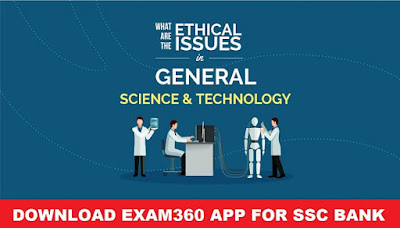# Science & Technology: Scientific Points for SSC Exams## Science & Technology Scientific Points

Here we are trying to find out some scientifc points of SCIENCE & TECHNOLOGY based on Previous Years Questions for Upcoming SSC CHSL & CGL 2018 Exams. These Points will help you to boost your score in SSC CGL 2018 & CHSL as well as Railway.
• If a hole is bored along a diameter of the earth and a stone is dropped into the hole, it will executive the S.H.M about the center of the earth.
• If a simple pendulum is vibrating in an evacuated chamber, it will oscillate for ever with the same amplitude and frequency.
• The length of second’s pendulum on surface of the moon, where g is 1/6th of the value of g on the surface of the earth is 1/6 m.
• A girl is swigging on a swing in the sitting position. The period of swing will now be shorter if she stands up.
• A simple harmonic oscillator has time period T. The time taken by it to travel from the extreme position to half the amplitude is T/6.
• A car is running on a road at uniform speed of 60 Km/h. The net resultant force on the car is equal to zero.
• The mass of a body on earth is 100 Kg (acceleration due to gravity, g = 10 m/s^2). If acceleration due to gravity on the moon is g/6, then mass of the body on moon is 100 Kg.
• The working principle of a washing machine is centrifugation.
• A simple pendulum has a time period T1 when on earth’s surface and T2 when taken to height R above earth’s surface, where R is the radius of the earth. The value of T2/T1 is 2.
• The young’s modulus of a perfectly rigid body is infinite.
• The modulus of rigidity of a liquid is 0.
• It is easier to swim in sea water than in ordinary water as density of sea water is higher than that of ordinary water.
• The pressure at the bottom of a liquid tank does not depend on area of the liquid surface.
• A piece of ice has a stone in it and floats in a vessel containing water. When the ice melts, the level of water in the vessel would fall.
• The operating principle of a hydraulic press is Pascal’s principle.
• The pressure of the earth’s atmosphere at sea level is due to the gravitational attraction of the earth atmosphere at sea level is due to the gravitational attraction of the earth for the atmosphere.
• An iron piece and a wooden piece have equal weights in air. If they are removed from air to vacuum the wooden piece appears to weigh more.

• A floating body always displaces liquid equal to its own weight.
• Two pieces of different metals when completely immersed in water experience equal upthrust then both pieces have equal volume.
• For a body float in liquid with some portion outside, the weight of the liquid displaced by the fully immersed body should be more than the weight of the body.
• A block of ice is floating in a glass containing water. When the ice melts complete, the level of water of the glass will not change.
• A steel ball is floating in beaker containing mercury. If some water is poured in the beaker the steel ball will move up.
• A bird is sitting in a wire cage which is heated from a spring balance , if the bird starts flying inside the cage, the reading of the spring balance be less than earlier.
• The shape of rain drop is spherical due to surface tension.
• In order that a floating object be in stable equilibrium, its center of buoyancy should be vertically above the center of gravity.
• A body is just floating in a liquid if the body is slightly pressure down and released it will sink to the bottom.
• Bernoulli’s Theorem is applicable to the flow of liquids.
• Bernoulli’s principle does not explain lift of a jet.
• An ideal liquid flows through a horizontal tube of variable diameter. The pressure is lowest where the velocity is highest.
• Dynamic Lift is related to Bernoulli’s Theorem.
• When a fluid passes through the constricted part of a pipe, its velocity increase and pressure decreases.
• The rate of leak from a hole in a tank is more if situated near the bottom.
• If a stream of air is blown under one of the pans of a physical balance in equilibrium, then the pan will go down.
• An application to the Bernoulli’s equation for fluid flow is found in dynamic lit of an aeroplane.
• Viscosity is the property of liquids by virtue of which they oppose the relative motion of its parts.
• The velocity of rain drop attains constant value because of viscous force exerted by air.
• If temperature rises, the co-efficient of viscosity of liquid decreases.
• A small and a large rain drops are falling through air, the large drop move faster.
• A liquid drop attains spherical shape due to surface tension.
• When some detergent is added to water, surface tension reduces.
• A liquid will not wet the surface if the angle of contact is obtuse.
• The tendency of a liquid drops to contract and occupy minimum area due to surface tension.